# Non-linear stability of numerical methods

Numerical stability theory for the initial value problem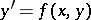,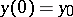, where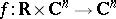, is concerned with the question of whether the numerical discretization inherits the dynamic properties of the differential equation. Stability concepts are usually based on structural assumptions on. For non-linear problems, the breakthrough was achieved by G. Dahlquist in his seminal paper [a3]. There, he studied multi-step discretizations of problems satisfying a one-sided Lipschitz condition. Letdenote an inner product onand letbe the induced norm.

## Runge–Kutta methods.

An-stage Runge–Kutta discretization of,is given by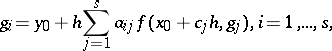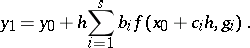Here,denotes the step-size and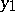is the Runge–Kutta approximation to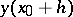. (For a thorough discussion of such methods, see [a1], [a2], and [a4]; see also Runge–Kutta method.)

### B-stability.

If the problem satisfies the global contractivity condition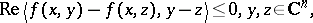then the difference of two solutions is a non-increasing function of. Let,denote the numerical solutions after one step of sizewith initial values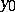,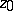, respectively. A Runge–Kutta method is called B-stable (or sometimes BN-stable), if the contractivity condition implies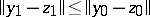for all. Examples of B-stable Runge–Kutta methods are given below. The definition of B-stability extends to arbitrary one-step methods in an obvious way.

### Algebraic stability.

A Runge–Kutta method is called algebraically stable if its coefficients satisfy

i)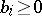,;

ii)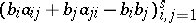is positive semi-definite. Algebraic stability plays an important role in the theory of B-convergence. Note that algebraic stability implies B-stability. For non-confluent methods, i.e.for, both concepts are equivalent. The following families of implicit Runge–Kutta methods are algebraically stable and therefore B-stable: Gauss, RadauIA, RadauIIA, LobattoIIIC.

### Error growth function.

Let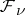(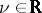) denote the class of all problems satisfying the one-sided Lipschitz condition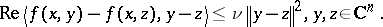For, this condition is weaker than contractivity and allows trajectories to expand with increasing. For any given real numberand step-size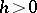, set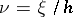and denote by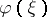the smallest number for which the estimate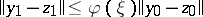holds for all problems in. The functionis called error growth function. For B-stable Runge–Kutta methods, the error growth function is superexponential, i.e. satisfies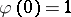and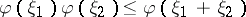for all,having the same sign. This result can be used in the asymptotic stability analysis of Runge–Kutta methods, see [a5].

## Linear multi-step methods.

A linear multi-step discretization ofis given by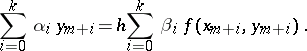Let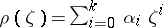andbe the generating polynomials. Using the normalization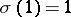, the associated one-leg method is defined by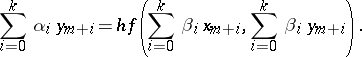(For a thorough discussion, see [a4].) A one-leg method is called G-stable if there exists a real symmetric positive-definite-dimensional matrixsuch that any two numerical solutions satisfy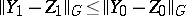for all step-sizes, whenever the problem is contractive (). Here,and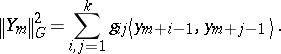G-stability is closely related to linear stability: If the generating polynomials have no common divisor, then the multi-step method is A-stable if and only if the corresponding one-leg method is G-stable. Thus, the-step BDF method is G-stable. There is also a purely algebraic condition that implies G-stability.

The concepts of G-stability and algebraic stability have been successfully extended to general linear methods, see [a1] and [a4].

Notwithstanding the merits of B- and G-stability, contractive problems have quite simple dynamics. Other classes of problems have been considered that admit a more complex behaviour. For a review, see [a7].

The long-time behaviour of time discretizations of non-linear evolution equations is an active field of research at the moment (1998). Basically, two different approaches exist for the analysis of numerical stability:

a) energy estimates;

b) estimates for the linear problem, combined with perturbation techniques. Whereas energy estimates require algebraic stability on the part of the methods, linear stability (A()-stability) is sufficient for the second approach. (For an illustration of these techniques in connection with convergence, see [a6].) Both approaches offer their merits. The latter, however, is in particular important for methods that are not B-stable, e.g., for linearly implicit Runge–Kutta methods.